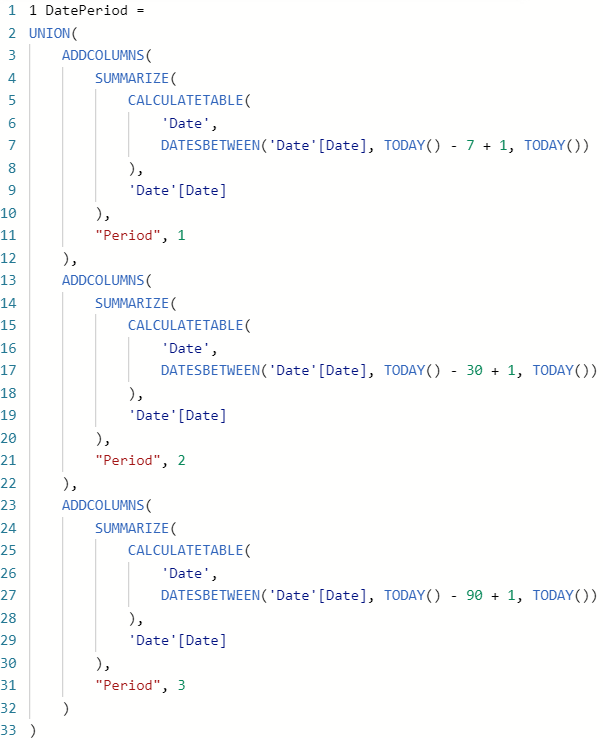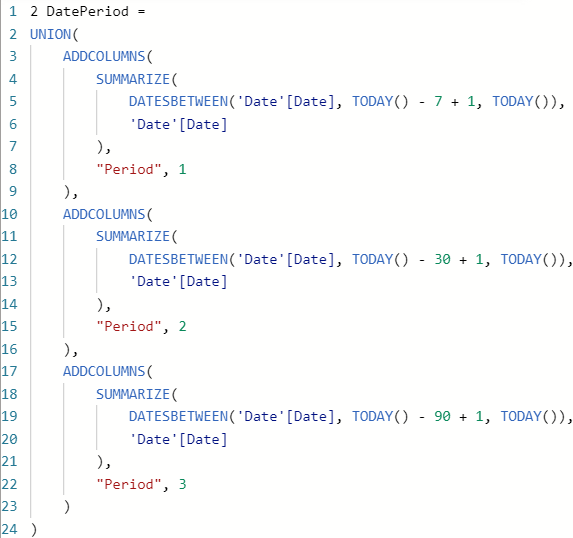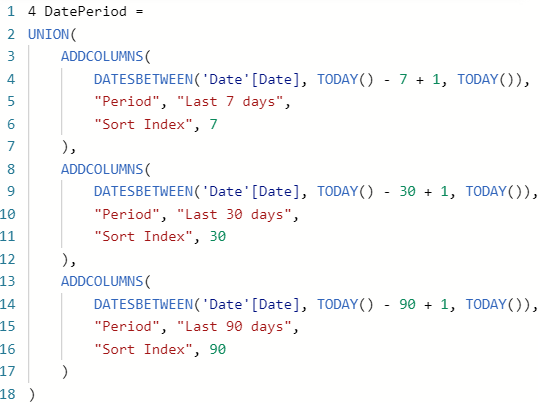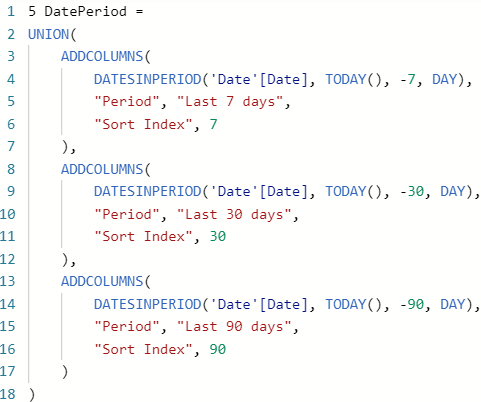# Power BI Antipatterns #3: Table functions

Do you think Microsoft’s own files always follow best practices? Think again! In this episode, I’m dissecting a calculated table DAX formula from a system file generated automatically in Power BI service.

Today’s formula comes from the automatically generated data protection metrics PBIX file, which you’ll have if your organization uses data protection metrics. Here’s the formula:At over 30 lines, overall, it’s a UNION of three tables, and each differs only by the second DATESBETWEEN parameter and a different Period number.

There’s also the following calculated column in the same table:

``````Sort Index =
IF( [Period] = 1, "Last 7 days",
IF( [Period] = 2, "Last 30 days",
IF( [Period] = 3, "Last 90 days"
)))``````

Note: don’t you think that the Sort Index column is actually Period, and Period is actually Sort Index? While I don’t know for sure, my guess on why these two columns were named in this way is because you wouldn’t be able to sort text values in a calculated column by an existing numeric column, if text values were derived from the numeric column. Read more about it here: Sort by Column in Power BI.

There are several issues with the calculated table formula:

1. Given that SUMMARIZE only needs the Date column from the Date table, why use CALCULATETABLE? DATESBETWEEN will give you a one-column Date table anyway.
2. Why do you need to use SUMMARIZE on the Date table, when you summarize by the Date column? There’s no change in grain, so you’re only selecting a column without summarizing anything. And when there’s just one column, there’s no need to select it.
3. Why don’t you include Sort Index in the table, so you don’t have to add a calculated column? Both ADDCOLUMNS and SELECTCOLUMNS can add more than one column at the same time.
4. Within each DATESBETWEEN, can we avoid the +1 weirdness and calling TODAY twice?
5. Do we really need to repeat most of the code for each table?

I’ll tackle these issues one by one.

### CALCULATETABLE not needed

If we get rid of CALCULATETABLE this is what our formula will look like:Already better, although we’ve got a long way to go.

### SUMMARIZE not needed

Now that we already have a one-column date table (courtesy of DATESBETWEEN), we don’t need SUMMARIZE anymore:Much better, isn’t it? We still don’t have the Sort Index column though, so we’ll add it next.

### Include Sort Index in the table

Instead of creating a calculated column, we can include the Sort Index column in the table formula. In the process, we can name columns properly and give the Sort Index more meaningful values (you’ll see why soon):While the formula got longer, now we don’t need a calculated column anymore.

### Clearer date ranges

Even though DATESBETWEEN does the job, we can make our code a bit clearer by using DATESINPERIOD. At the same time, we’ll only need to call TODAY once for each period:At the same time, we don’t need “+ 1” in each DATESINPERIOD, so it’s cleaner.

### Avoiding repeated code

Finally, if we don’t want to repeat any of our code, we need to adopt a different approach. Instead of UNION, we can use DATATABLE and GENERATE: the former will contain the periods, while the latter will allow us to write DATESINPERIOD only once:See how short the formula is? Admittedly, this is a big leap compared to the previous code changes, so I’ll explain it a bit.

DATATABLE is DAX’s equivalent of the Enter data functionality; DATATABLE won’t create an M query, which is preferable in our case because we don’t need the table with periods and sort indexes by itself — it’s only useful with dates.

Note how I used the Sort Index column in DATESINPERIOD: this way I only need to use DATESINPERIOD once, since GENERATE goes through our periods table row by row and for each row it generates a new date table; in the end all tables are combined, like in UNION, except we’re not using UNION.

Important: try not to use volatile functions, like TODAY, in calculated columns and calculated tables. More details: Understanding model recalculation in Power BI.